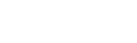# Peirce’s Criterion: Definition

## What is Peirce’s Criterion?

Peirce’s Criterion is a method, proposed by Benjamin Peerce in 1852, that allows us to eliminate outliers from data sets.

An outlier is a part of the data set that is abnormal and not representative of the general trend. Finding and eliminating outliers is an important part of much scientific research based on real-life measurements, and Peirce’s Criterion is one of the most theoretically rigorous ways to do this.

It’s also more generally applicable than other methods of eliminating outliers, including the more commonly used Chauvenet’s Criterion. It doesn’t make any arbitrary assumptions on the rejection of data. And, to make it even more useful, it can be used to eliminate more than one outlier.

## How to use Peirce’s Criterion

Using Peirce’s Criterion in its original form is cumbersome, but it has been condensed into a set of tables (see Chauvenet & Peirce, 2018) and an easy to use formula which can be easily applied to data sets.

R in these tables is a ratio: the ratio of the maximum allowable deviation from the sample set’s mean to the standard deviation. That is,Knowing R, then, we can calculate the value of |Xi – Xm| max from the standard deviation.

To use this modern form of Peirce’s Criterion, one would

1. Find the mean and sample standard deviation for the entire set.
2. Look up the value of R in a Peirce’s table that corresponds to the number of observations in your sample set. Begin by assuming one outlier, although you may repeat the process to discover more than one.
3. Use the formula |Xi – Xm| max= σ R to calculate the maximum allowable deviation.
4. Calculate the actual deviation of your potential outliers. |Xi – Xm|
5. Check if |Xi – Xm| > |Xi – Xm|max, and if it is, eliminate that outlier.
6. Now assume two outliers, and go through step 2-5 again. Keep the original number of measurements as well as the original values of the standard deviation and mean.
7. If your calculations in step 6 give you another outlier, you can repeat the process. Assume an additional outlier each time through and use the original number of measurements, mean and standard deviation each time.
8. Once all questionable data has been tested, calculate the mean and standard deviation again for your final data set.

## References

Jones, A. (2018). Probability, Statistics and Other Frightening Stuff. Routledge
Chauvenet, W. & Peirce, B. (2018). A Treatise On the Method of Least Squares: Or, the Application of the Theory of Probabilities in the Combination of Observations. Franklin Classics.

CITE THIS AS:
Stephanie Glen. "Peirce’s Criterion: Definition" From StatisticsHowTo.com: Elementary Statistics for the rest of us! https://www.statisticshowto.com/peirces-criterion/
---------------------------------------------------------------------------Need help with a homework or test question? With Chegg Study, you can get step-by-step solutions to your questions from an expert in the field. Your first 30 minutes with a Chegg tutor is free!